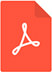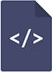# Tunneling transmission coefficient from the external electric field barrier

Open Access Journal of Science
Hasan Hüseyin ErbilPDF Full Text

# Abstract

According to classical physics theories, a moving particle cannot move to an environment with greater potential energy than its total energy during movement. But according to quantum theories, this event is well known. This event is called tunneling. Tunneling is a probability, and it is measured by a transmission coefficient. Correct calculation of this coefficient is very important because very sensitive and important instruments have been developed based on this event, and many events in nature can be explained by tunneling. This coefficient is generally calculated by semi-classical approaches (WKB) and the known formula is an approximate formula. In previous publications, the general transmission coefficient of a potential barrier with arbitrary form is calculated by a simple method without any approximation. The results are applied to calculate the half-life values of the nuclei that emit alpha particles. The half-life values obtained from our calculations and the classical method (WKB) have been compared, and it has been found that the new halflife values are exactly consistent with the experimental values. In this article, this general transmission coefficient is applied to calculate transmission coefficient for an arbitrary form external electrical field barrier (cold emission by the metal). The values obtained from our calculations and those of classical method (WKB) have been compared.

# Keywords

tunneling, transmission coefficient, cold emission by metal, barrier of electrical field